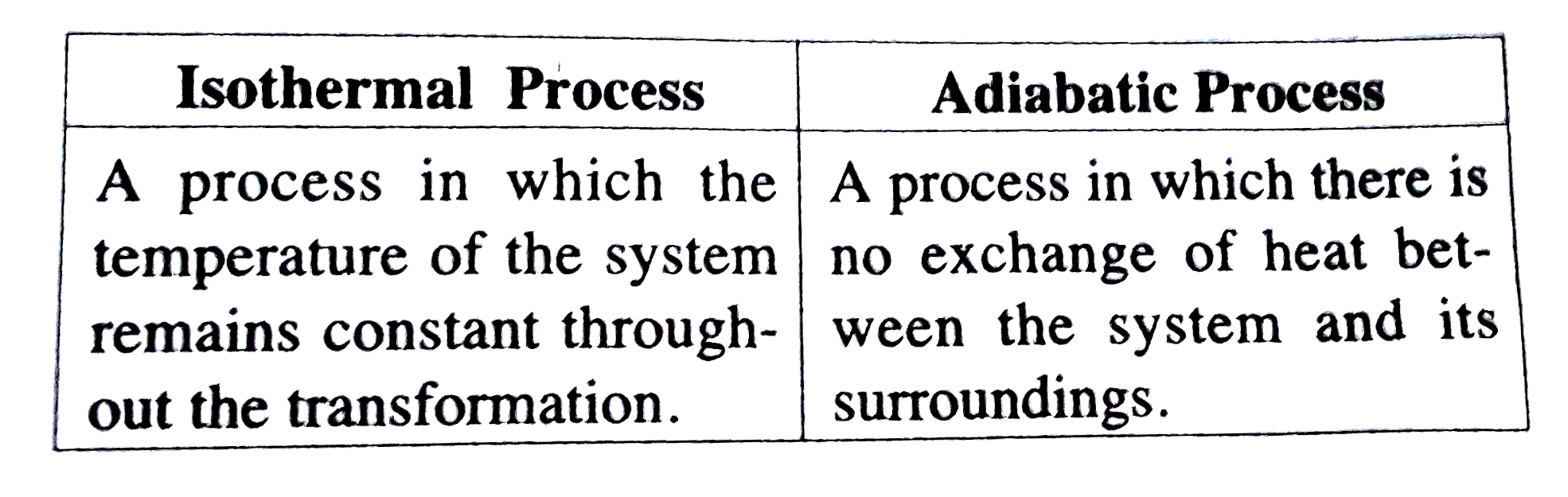# An organs substance (M=169 gram "mol"^(-1)) is dissolved in 2000 "cm^(3) of water. Its osmotic pressure at 12^(@)C was found to be 0*54atm. If

49 views

closed
An organs substance (M=169 gram "mol"^(-1)) is dissolved in 2000 "cm^(3) of water. Its osmotic pressure at 12^(@)C was found to be 0*54atm. If R=0*0821L atm. K^(-1) mol^(-1), calculate the mass of the solute.
Calculate the number of atoms in a unit cell of a metal crystalling in face centered cubic structure.
Distinguish between isothermal process and adiabatic process.
Mention the names of various steps involved in the extraction of pure metals from their ores.

by (91.7k points)
selected

M=169 gm /mol
Vol. of solvent =200 cm^(3)=2L
pi=0-54
T=12^(@)C=12+273=285K
R=0*0821L atm K^(-) "mol"^(-1)
m=?
pi V= n RT
0*54xx2=(m)/(169)xx0*-082xx285
m=(0*54xx2xx169)/(0*082xx285)
=(182*52)/(23*37)=7*8g
No. of atoms situated at 8 corners of unit cell of fcc type
(1)/(8)xx8=1
No. of atoms on each sphere
=(1)/(2)
Total no. of sphere present in each faces of unit cell
(1)/(2)xx6=3
Thus, total spheres present in fcc type =1+3=4Various stpes involved in the extraction of pure metals from their ores are :
(a) Concentration of ores.
(b) Conversion of ores into oxides.
(c) Reduction of ores to form crude metals.
(d) Refining of metals.